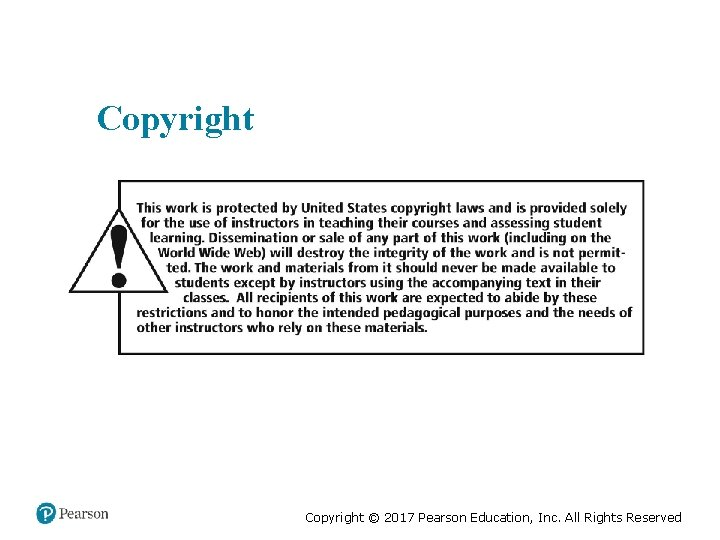# Exploring Microsoft Excel 2016 Comprehensive First Edition Chapter

• Slides: 27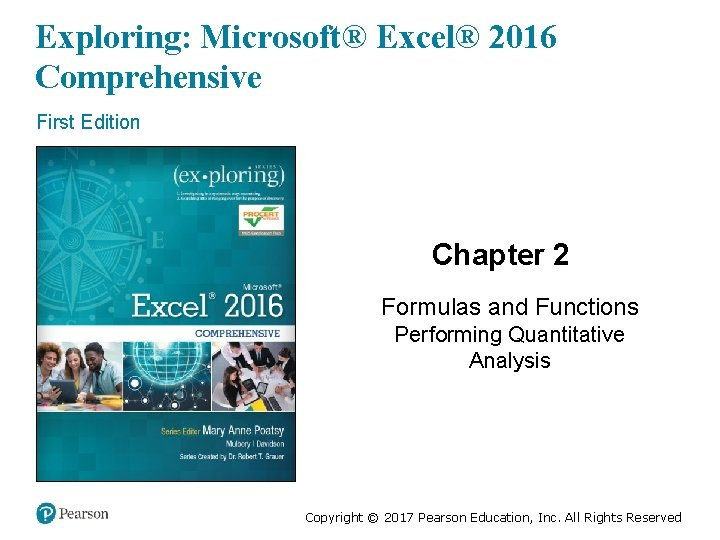Objectives • Use Relative, Absolute, and Mixed Cell References in Formulas • Insert a Function • Insert Basic Math and Statistics Functions • Use Date Functions • Determine Results with the IF Function • Use Lookup Functions • Calculate Payments with the PMT function Copyright © 2017 Pearson Education, Inc. All Rights ReservedObjective 1: Use Relative, Absolute, and Mixed Cell References in Formulas Skills: • Use a Relative Cell Reference • Use an Absolute Cell Reference • Use a Mixed Cell Reference Copyright © 2017 Pearson Education, Inc. All Rights ReservedUse Relative, Absolute, and Mixed Cell References in Formulas (1 of 4) • Relative cell reference—default method of referencing • Absolute cell reference—provides a constant reference to a specific cell • Mixed cell reference—combines absolute and relative cell references • F 4—toggles through relative, absolute, and mixed references Copyright © 2017 Pearson Education, Inc. All Rights Reserved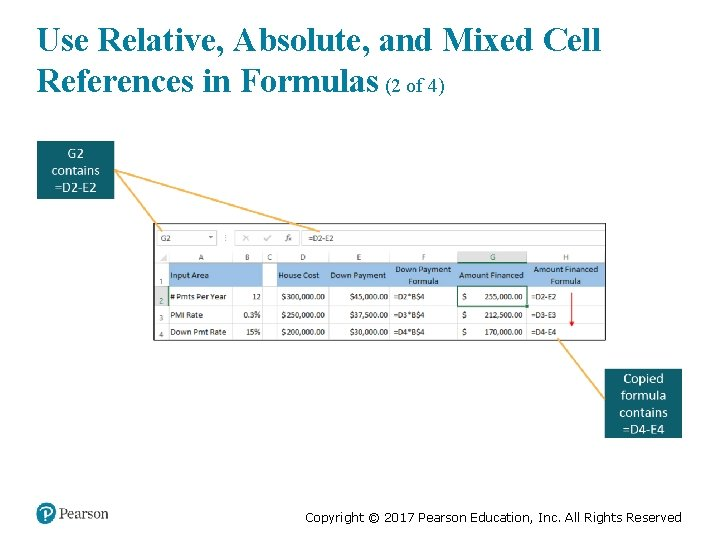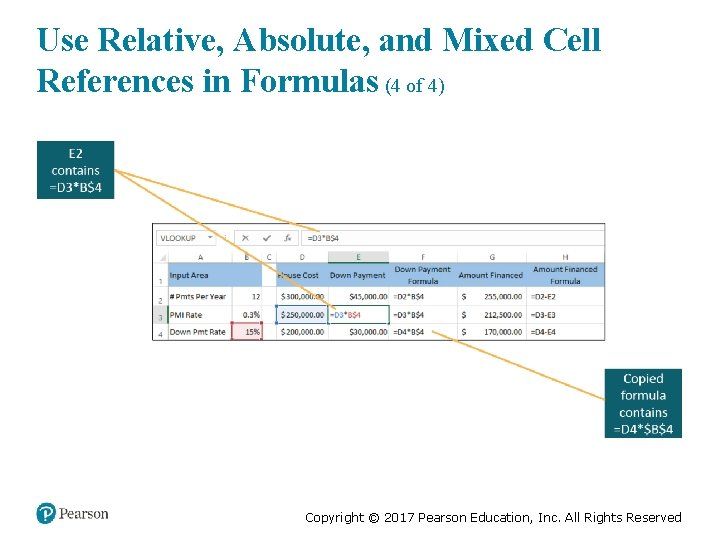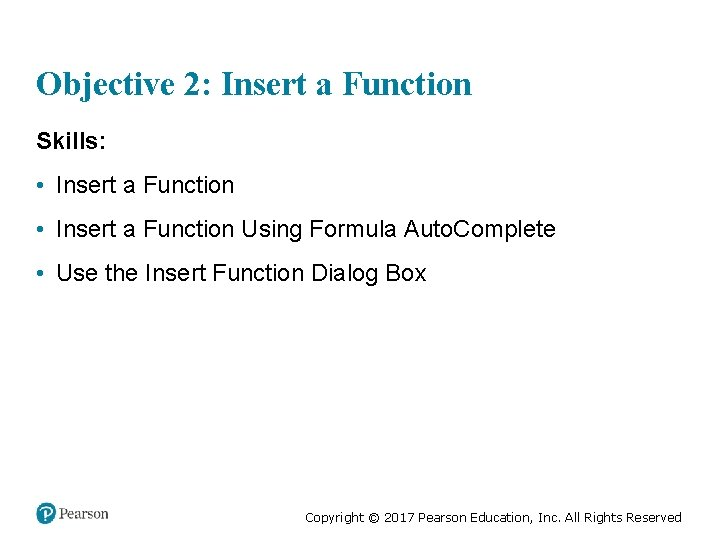Objective 2: Insert a Function Skills: • Insert a Function Using Formula Auto. Complete • Use the Insert Function Dialog Box Copyright © 2017 Pearson Education, Inc. All Rights Reserved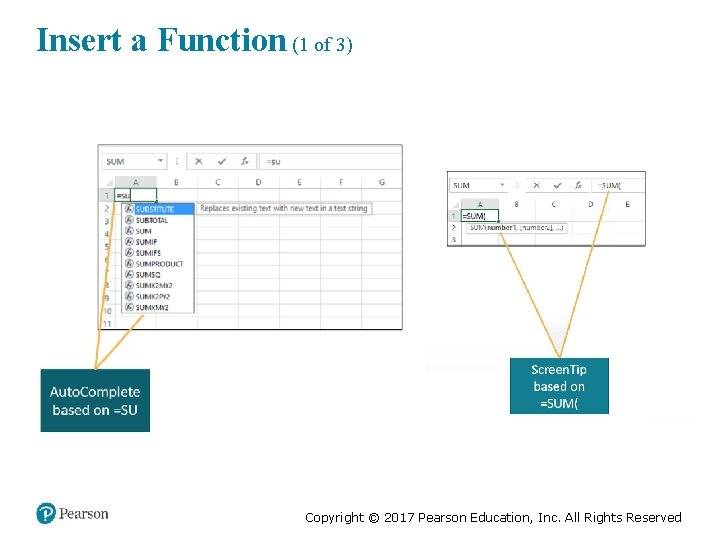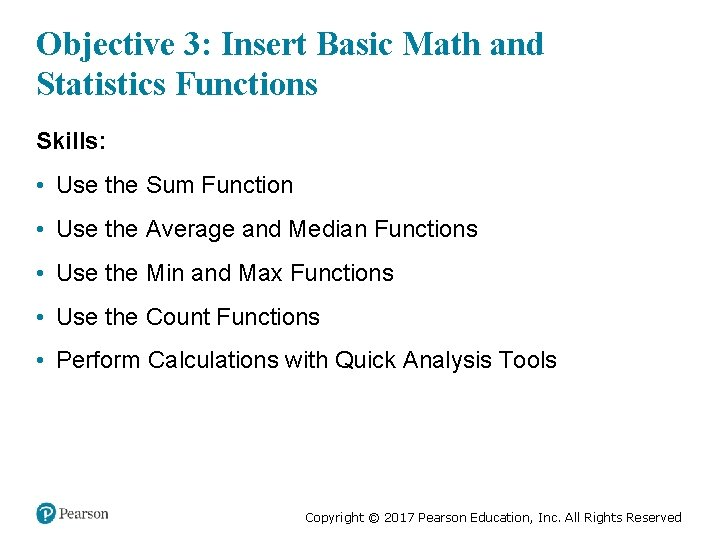Objective 3: Insert Basic Math and Statistics Functions Skills: • Use the Sum Function • Use the Average and Median Functions • Use the Min and Max Functions • Use the Count Functions • Perform Calculations with Quick Analysis Tools Copyright © 2017 Pearson Education, Inc. All Rights ReservedInsert Basic Math and Statistics Functions (1 of 2) • =SUM(number 1, [number 2], …) – =SUM(A 1, A 2, A 5) • =AVERAGE(number 1, [number 2], …) – =AVERAGE(B 10: B 20) • =MEDIAN(number 1, [number 2], …) – =MEDIAN(A 2: A 14, F 2: F 14) • =MIN(number 1, [number 2], …) – =MIN(A 1, B 10: C 25) Copyright © 2017 Pearson Education, Inc. All Rights Reserved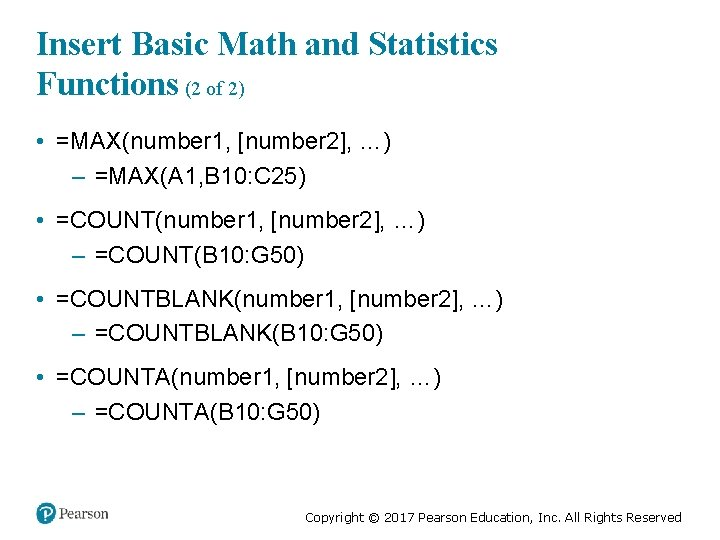Insert Basic Math and Statistics Functions (2 of 2) • =MAX(number 1, [number 2], …) – =MAX(A 1, B 10: C 25) • =COUNT(number 1, [number 2], …) – =COUNT(B 10: G 50) • =COUNTBLANK(number 1, [number 2], …) – =COUNTBLANK(B 10: G 50) • =COUNTA(number 1, [number 2], …) – =COUNTA(B 10: G 50) Copyright © 2017 Pearson Education, Inc. All Rights Reserved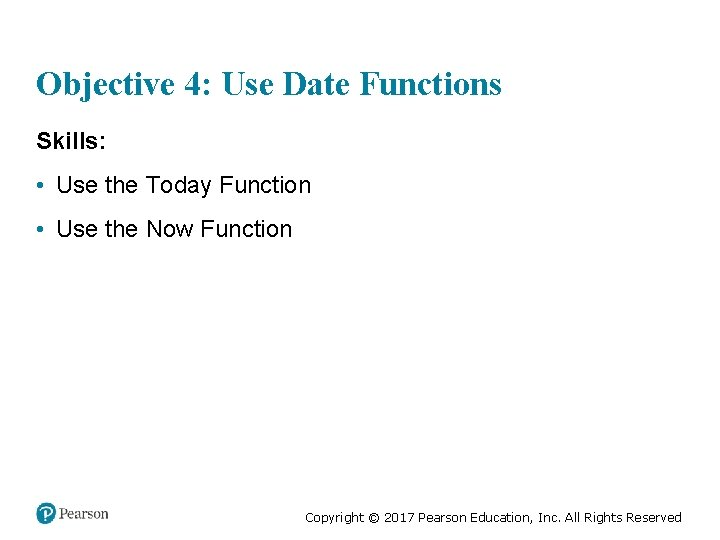Use Date Functions • =TODAY() – Displays a result like: 2/9/2018 • =NOW() – Displays a result like: 2/9/2018 14: 09 Copyright © 2017 Pearson Education, Inc. All Rights Reserved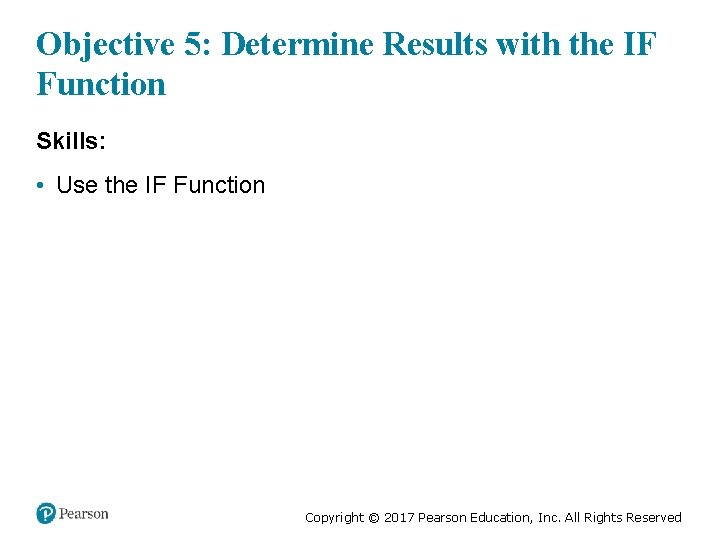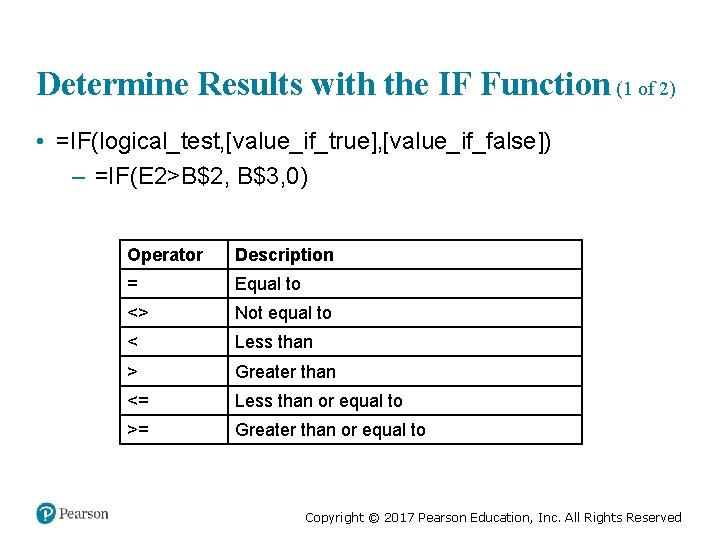Determine Results with the IF Function (1 of 2) • =IF(logical_test, [value_if_true], [value_if_false]) – =IF(E 2>B\$2, B\$3, 0) Operator Description = Equal to <> Not equal to < Less than > Greater than <= Less than or equal to >= Greater than or equal to Copyright © 2017 Pearson Education, Inc. All Rights Reserved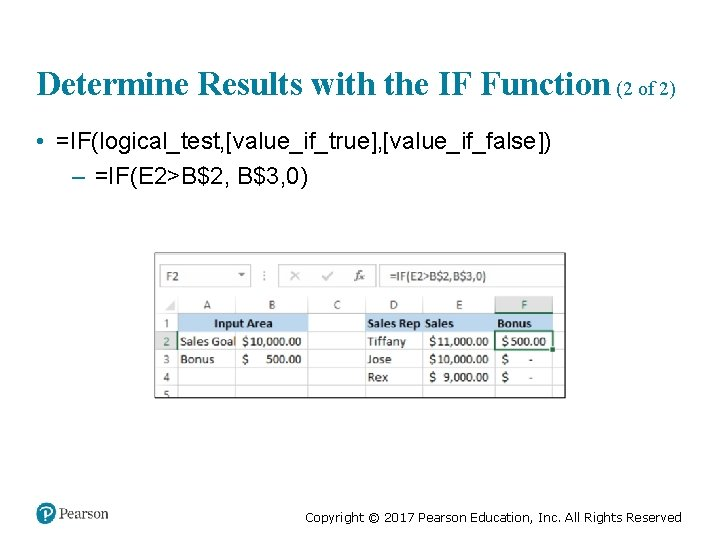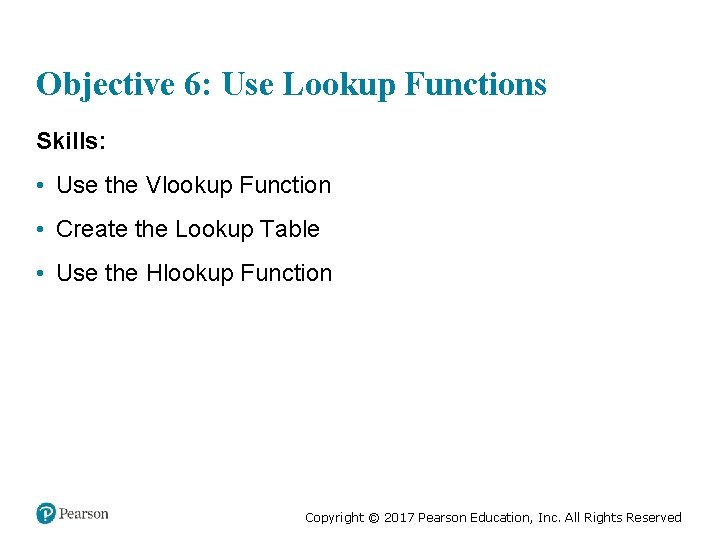Objective 6: Use Lookup Functions Skills: • Use the Vlookup Function • Create the Lookup Table • Use the Hlookup Function Copyright © 2017 Pearson Education, Inc. All Rights Reserved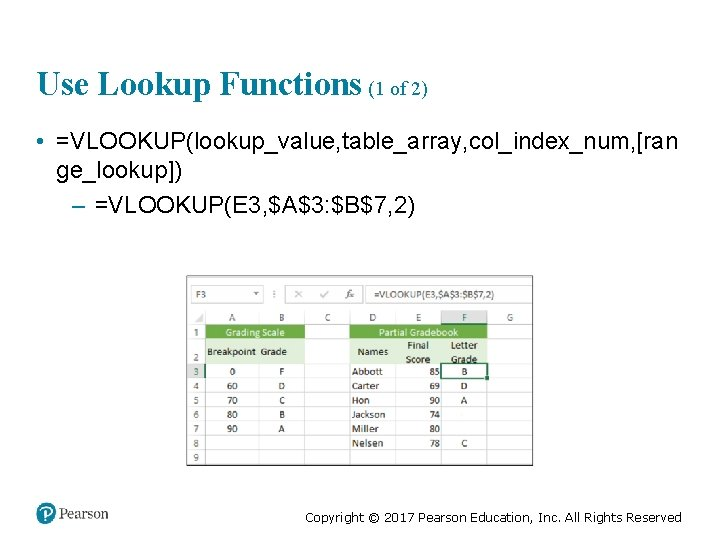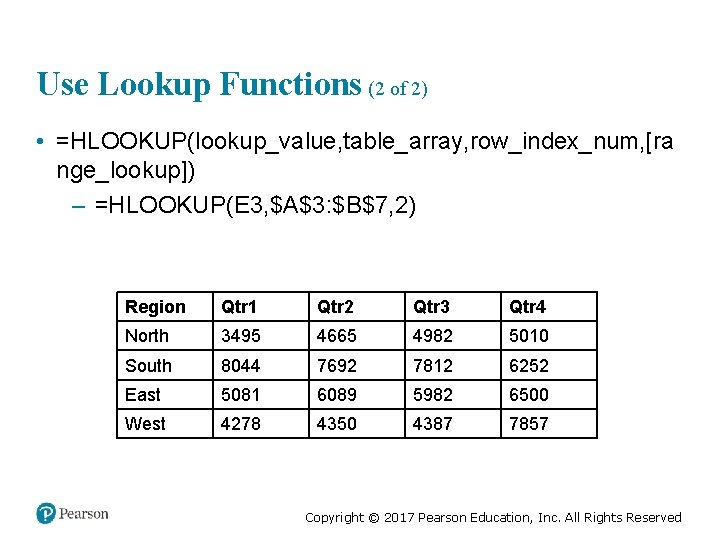Use Lookup Functions (2 of 2) • =HLOOKUP(lookup_value, table_array, row_index_num, [ra nge_lookup]) – =HLOOKUP(E 3, \$A\$3: \$B\$7, 2) Region Qtr 1 Qtr 2 Qtr 3 Qtr 4 North 3495 4665 4982 5010 South 8044 7692 7812 6252 East 5081 6089 5982 6500 West 4278 4350 4387 7857 Copyright © 2017 Pearson Education, Inc. All Rights Reserved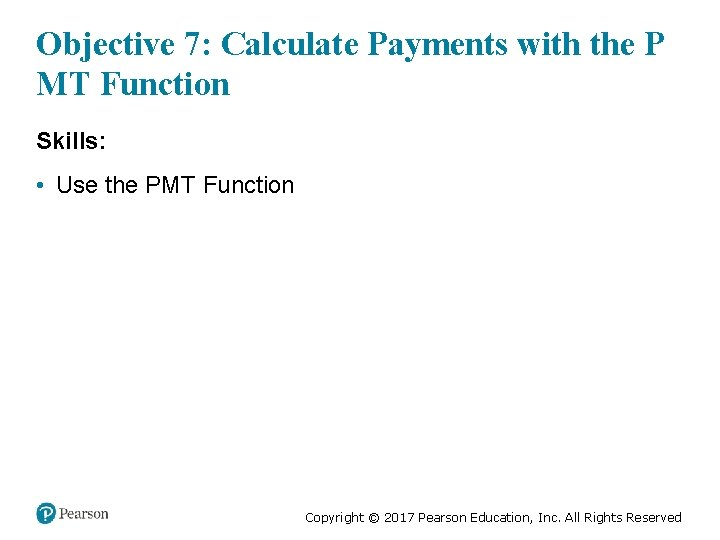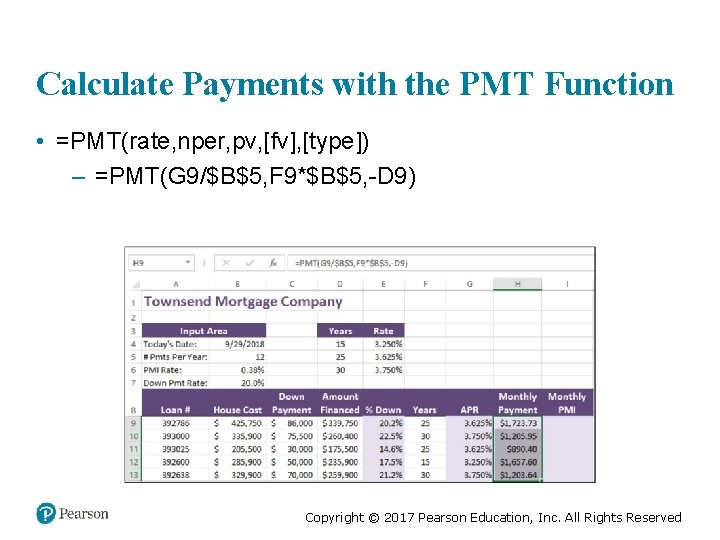Calculate Payments with the PMT Function • =PMT(rate, nper, pv, [fv], [type]) – =PMT(G 9/\$B\$5, F 9*\$B\$5, -D 9) Copyright © 2017 Pearson Education, Inc. All Rights Reserved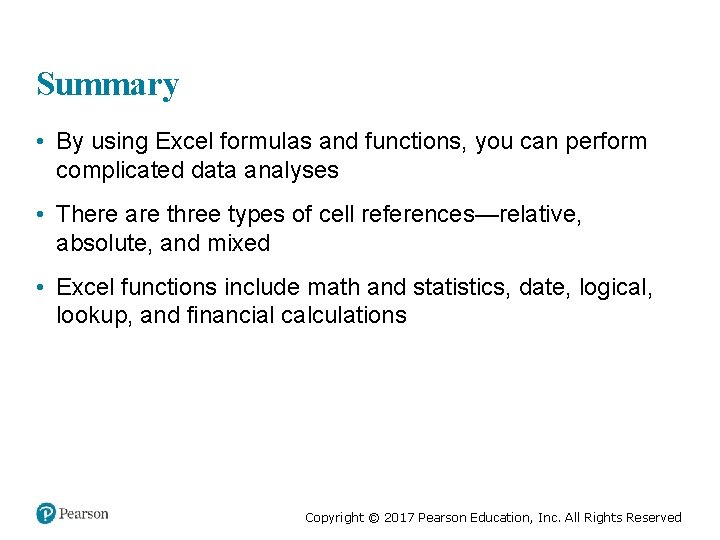Summary • By using Excel formulas and functions, you can perform complicated data analyses • There are three types of cell references—relative, absolute, and mixed • Excel functions include math and statistics, date, logical, lookup, and financial calculations Copyright © 2017 Pearson Education, Inc. All Rights Reserved